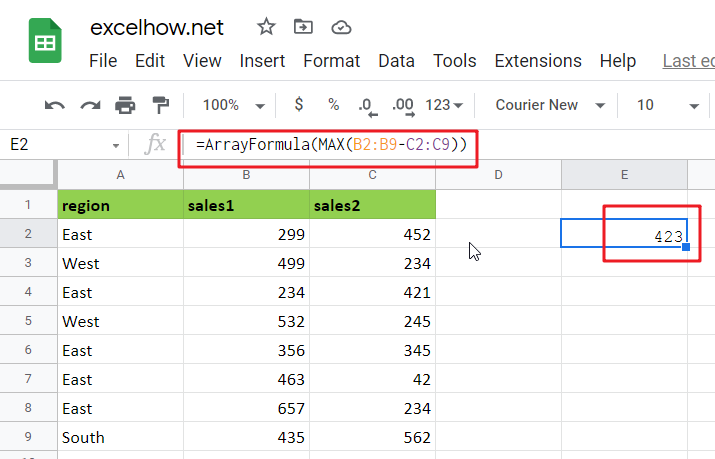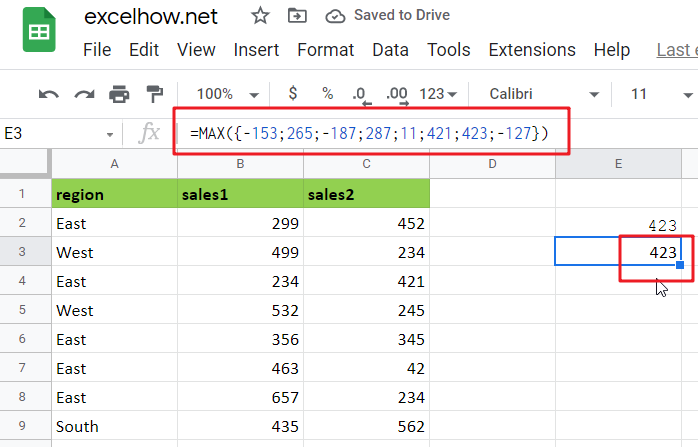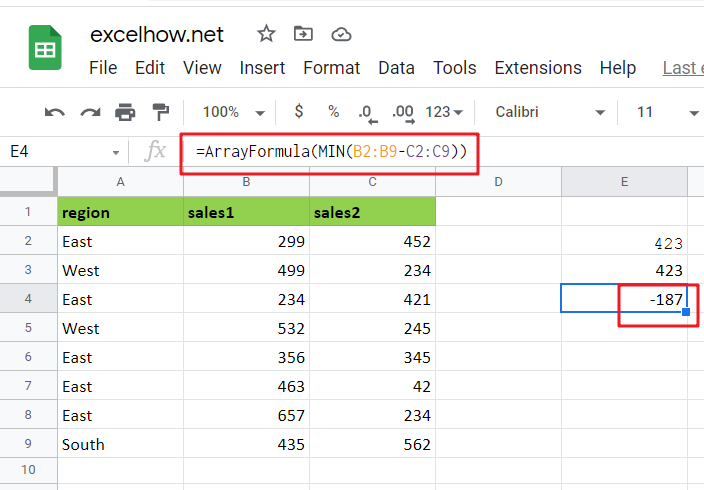# Basic Array Formula With Examples in Google Sheets

Are you searching for an article for getting the basic array formula with different examples for better understanding? Then congratulations because you have just landed on the right article. In this article, you would get to know the basic array formula in google sheets and the use of this formula in different examples with a brief explanation.

### General Formula in Google Sheets

The basic array formula is given as follows:

{=MAX(range1-range2)}

### Syntax Explanation

• Minus Operator (-): This symbol is used to subtract any two values.
• Parenthesis (): The main function of this symbol is to group the elements.
• range1: This is the first input range in the Google sheet.
• Range2: This refers to the second input range.
• MAX: The MAX Function returns the largest numeric value from the range of input values.
• MIN: The MIN Function returns the smallest numeric value from a range of input values.

## Explanation

In Google Sheets, an Array Formula allows you to perform complex calculations on one or more value sets. The result could be a single cell or an array. An array is simply a list or range of values, but an Array Formula is a particular type of formula that must be entered by pressing Ctrl+Shift+Enter after that, the formula would be displayed in the formula bar surrounded by curly brackets {=…}

Array formulas are commonly used for data analysis, conditional sums and lookups, linear algebra, matrix math and manipulation, and many other tasks. A new Google Sheets user may come across array formulas in other people’s spreadsheets, but creating array formulas is typically an intermediate-to-advanced topic.

An array formula is commonly used to calculate the maximum or minimum change in a data set without using a helper column. In the example, the formula in E2 is mentioned as follows:

`=MAX(B2:B9-C2:C9)`

#This array formula must be entered with control + shift + enter.

This page’s example shows a simple array formula. Working from the inside out, the expression:

=B2:B9-C2:C9

As a result, an array of seven values is returned:

`{-153;265;-187;287;11;421;423;-127}`

#Each number in the array results from subtracting the “low” from the “high” in each of the seven rows of data. This array is returned to the MAX function:

=MAX({-153;265;-187;287;11;421;423;-127})

#And MAX returns the maximum value in the array, which is 423.

To return the smallest change in the data, substitute the MIN function for the MAX function:

`=MIN(B2:B9-C2:C9)`

#As before, this is an array formula that must be entered with control + shift + enter.

## More Examples For Better Understanding in Google Sheets

For basic array formulas in Google Sheets, use the steps below.

In the following example, you must create a sample data set in Google Sheets.

Then, in the formula bar, use the following formula to count the maximum change in a data set.

=MAX(B2:B9-C2:C9)

After pressing Ctrl+Shift+Enter, you will see the MAX value in cell E2.

Then, in the formula bar, use the following formula to count the minimum change in a data set.

=MIN(B2:B9-C2:C9)

Finally, after pressing Ctrl+Shift+Enter, you will see the result for the MIN in cell F2.

## More On Array Formulas

To understand array formulas, you must first learn to investigate the results of various operations within a formula as Google Sheets evaluates it. You must understand how to use the F9 key to debug a formula and how to use Google Sheets’s Evaluate Feature.

## Summary

### Related Functions

The Google Sheets MIN function returns the smallest numeric value from the numbers that you provided. Or returns the smallest value in the array.The MIN function is a build-in function in Google Sheets and it is categorized as a Statistical Function.The syntax of the MIN function is as below:= MIN(num1,[num2,…numn])….
The Google Sheets MAX function returns the largest numeric value from the numbers that you provided. Or returns the largest value in the array.= MAX(num1,[num2,…numn])…

Related Posts

Cap percentage values between 0 and 100

This article will talk about how to cap the percentage values between 0% and 100% in Microsoft Excel Spreadsheet or Google Sheets. If you are a newbie on Excel or google Sheets, you may be able to do this by ...

Calculate Cap Percentages to Specific Value

This article will talk about how to limit the cap percentage of a given amount to a given value in Microsoft Excel Spreadsheet or Google Sheets. If you are a newbie on Excel or google Sheets, you may be able ...

Find the Closest Data to the Data Provided in Google Sheets

In our daily work, we may encounter such an issue that to find the closest value to a certain value. In fact, google sheets internal functions can help us solve this problem. In today’s article, we will show you how ...

Average Of Numbers With Multiple Criteria in Google Sheets

Have you ever come across a task to calculate the average of the numbers with respect to multiple criteria in google sheets? Are you tired of doing this cumbersome task manually? Are you willing to do this task smartly in ...

Calculate Average Of Last 5 Or N Values In Columns in Google Sheets

Suppose you come across a task where you need to calculate the average of the last 2 or 3 numeric values in google sheets, then what would you do? If you are new to google sheets, then your first attempt ...

Sidebar